# 生成对抗网络——原理解释和数学推导

## 生成对抗网络系列文章（1）

Posted by Canary on May 5, 2018

## GAN的基本思想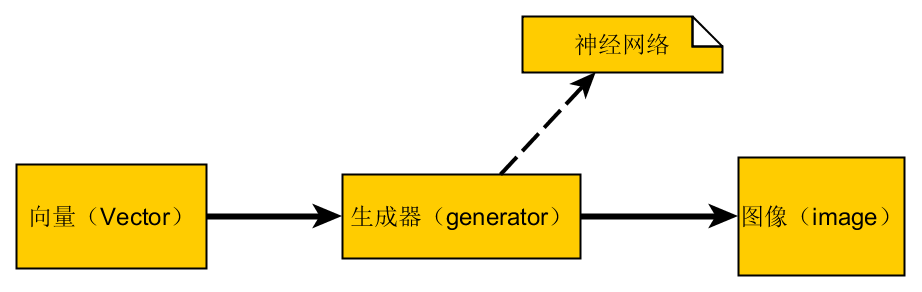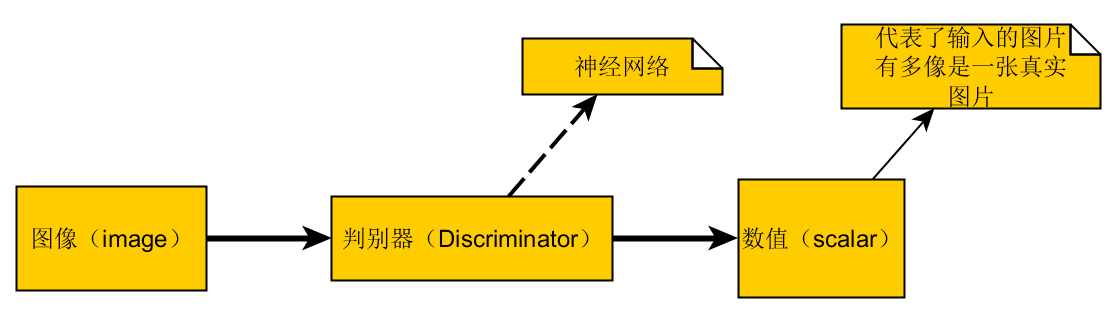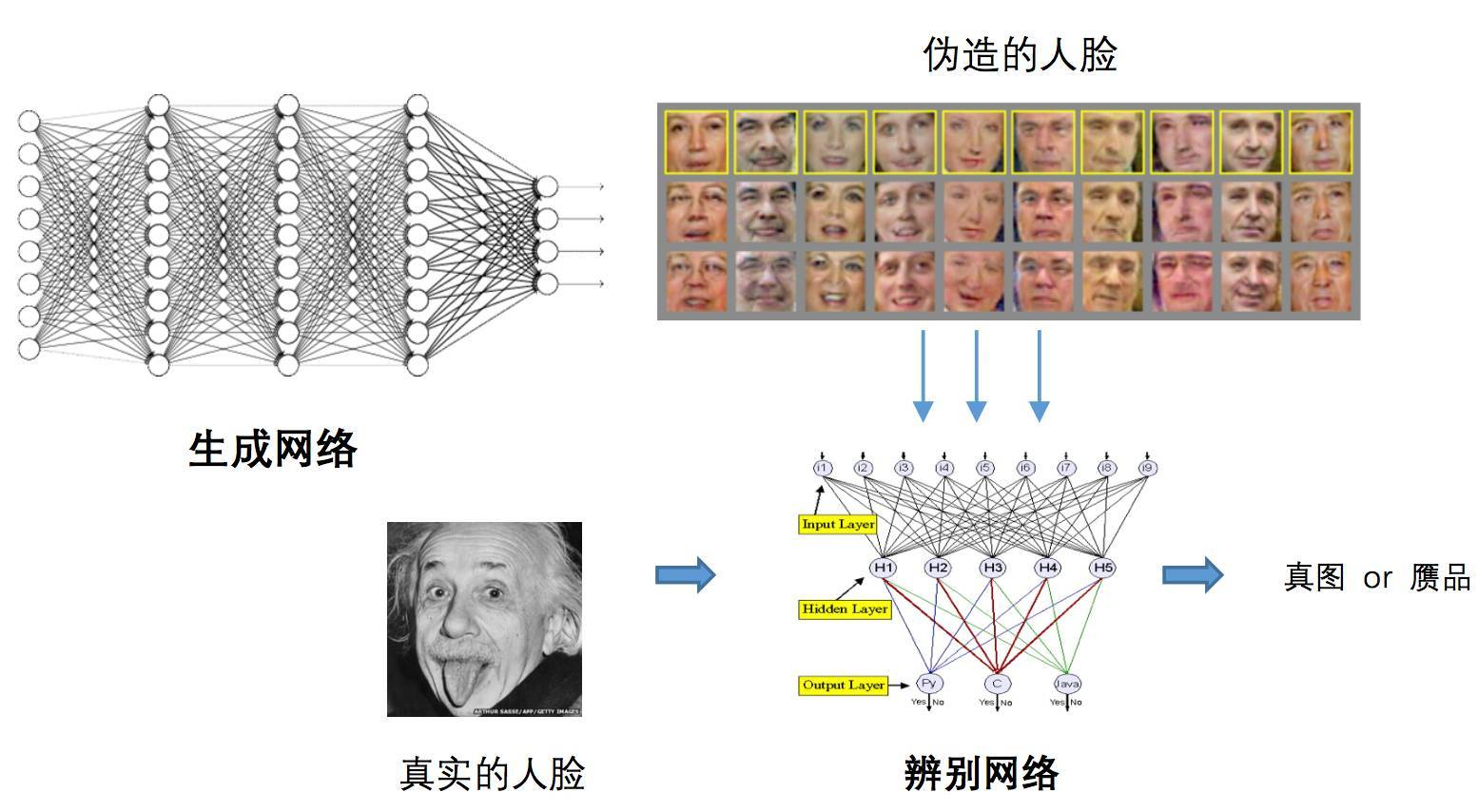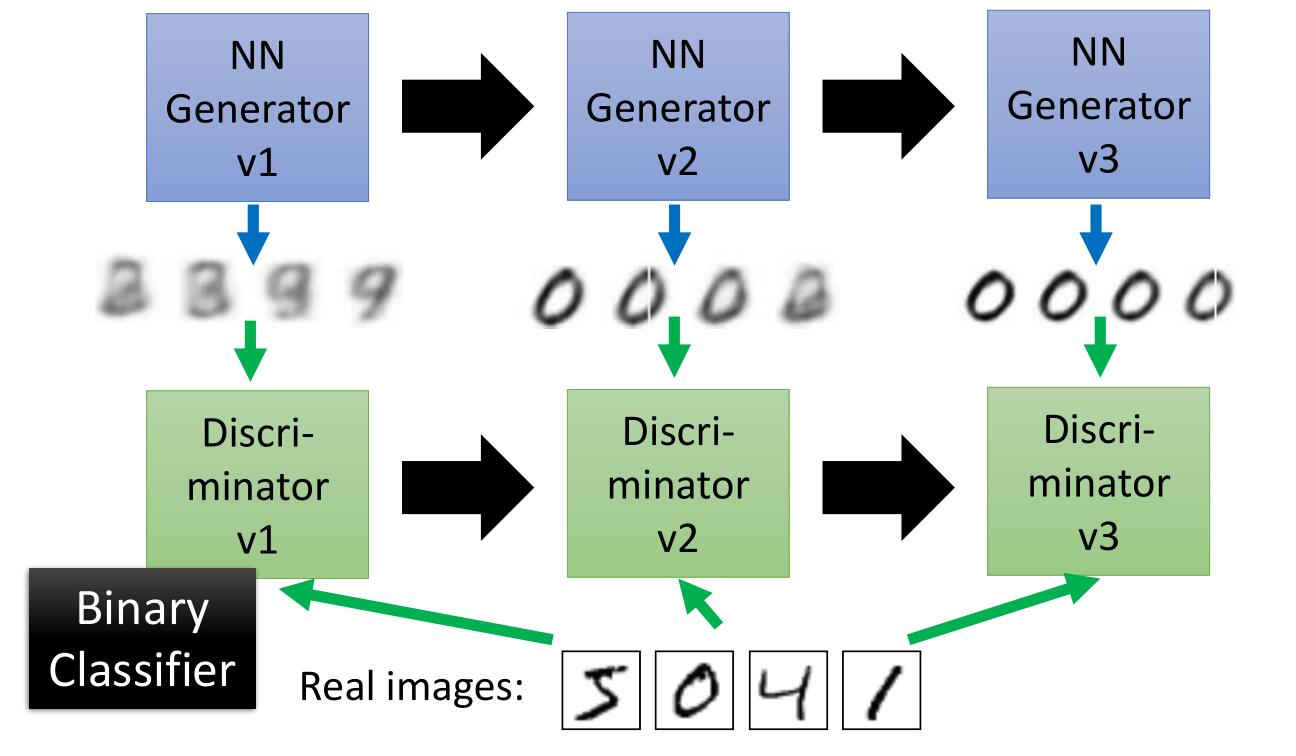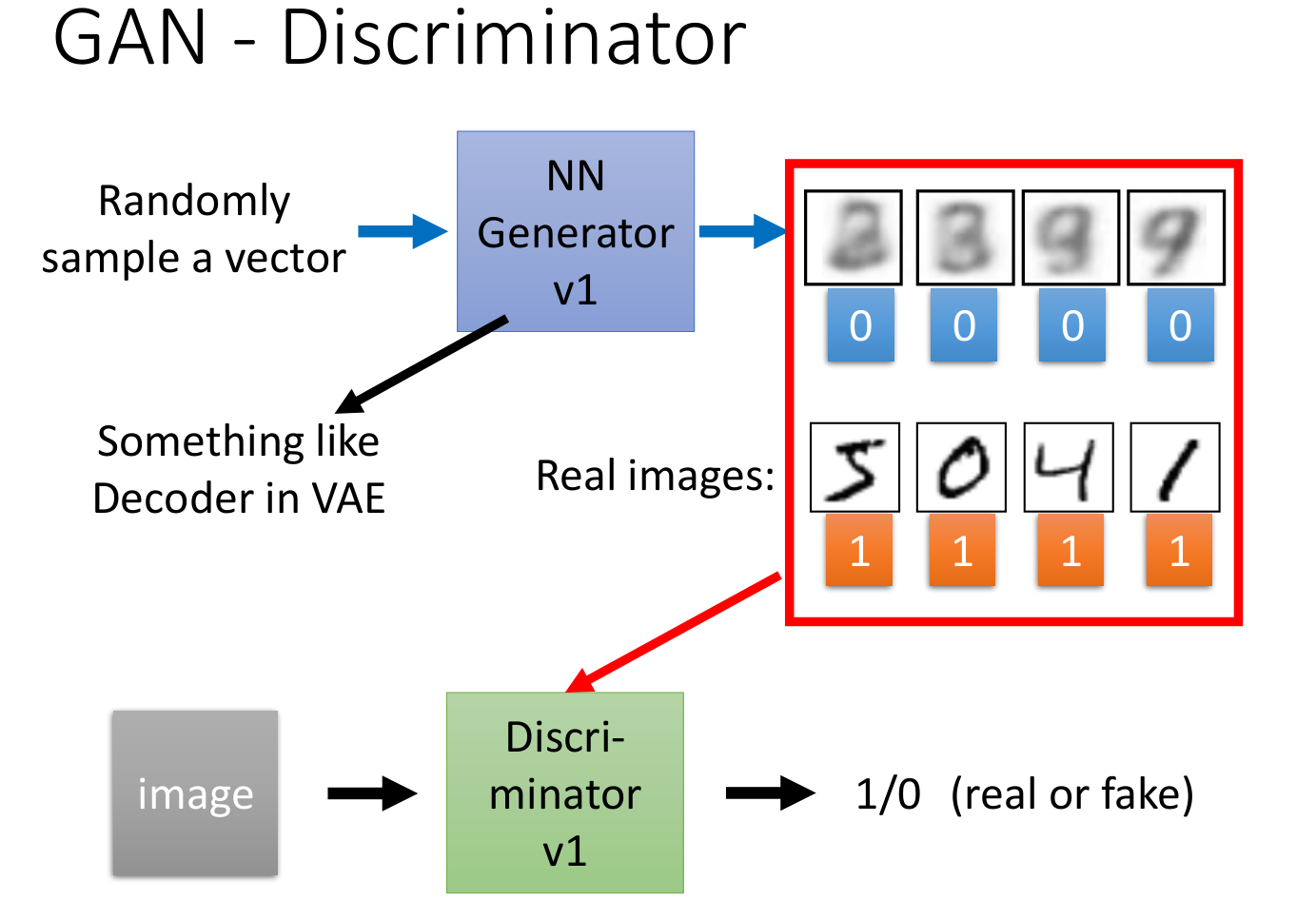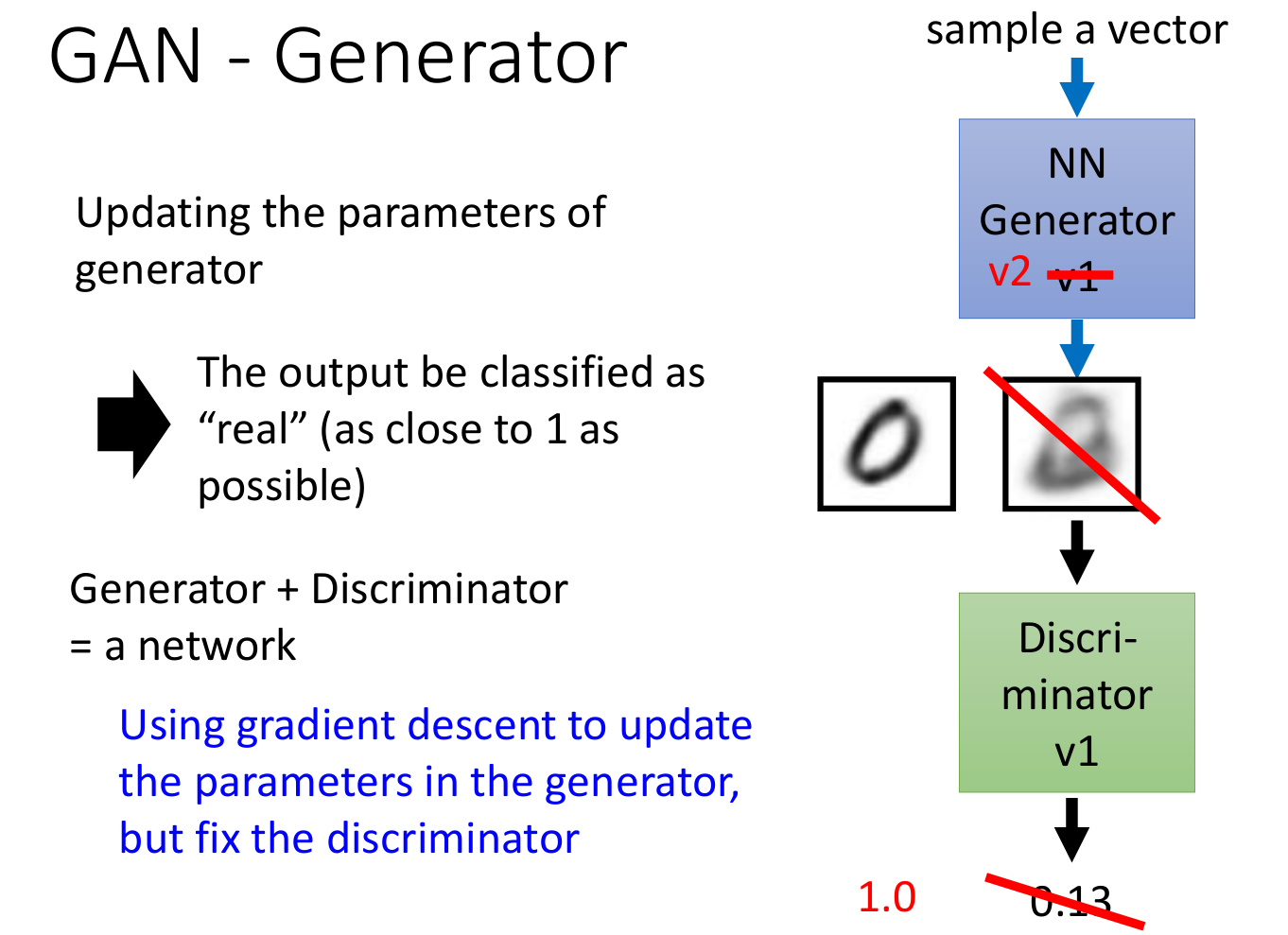## GAN推导的数学知识

#### KL散度

• 第1行照抄，不赘述；
• 第2行就是直接加上 $log$ 用来方便后边的运算，连乘变成连加；
• 第3行就是除以 $m$ 得到和训练数据 $X$ 的经验分布 $\hat p_{data}$ 相关的期望；
• 第4行就是把期望展开，后边减去的那一项是为了后边变形为KL散度做准备，这一项不会影响到 $θ_{ML}$ 的取值；
• 后边的就是简单的变形而已。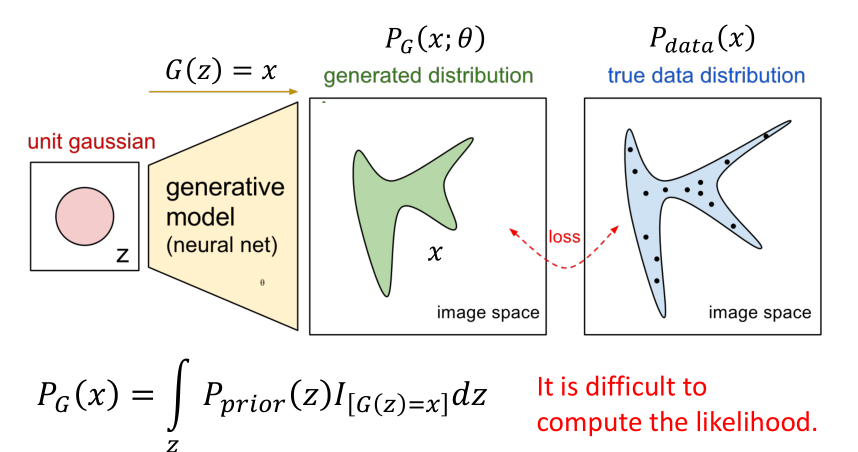#### JS散度

JS散度(Jensen-Shannon divergence)度量了两个概率分布的相似度，基于KL散度的变体，解决了KL散度非对称的问题。一般地，JS散度是对称的，其取值是 0 到 1 之间。定义如下：

KL散度和JS散度度量的时候有一个问题：如果两个分布离得很远，完全没有重叠的时候，那么KL散度值是没有意义的，而JS散度值是一个常数。这在学习算法中是比较致命的，这就意味这这一点的梯度为 0。梯度消失了。

## GAN算法推导

1. 生成器(Generator,G)
• Generator是一个函数，输入是 $z$ ，输出是 $x$ ；
• 给定一个先验分布 $p_{prior}(z)$ 和反映生成器G的分布 $P_G(x)$，$P_G(x)$ 对应的就是上一节的 $p_{model}(x;θ)$ ；
2. 判别器(Discriminator,D)
• Discriminator也是一个函数，输入是 $x$ ，输出是一个标量；
• 主要是评估 $P_G(x)$ 和 $P_{data}(x)$ 之间到底有多不同，也就是求他们之间的交叉熵，$P_{data}(x)$ 对应的是上一节的 $p_{data}(x)$。

#### 寻找最好的 $D^{* }$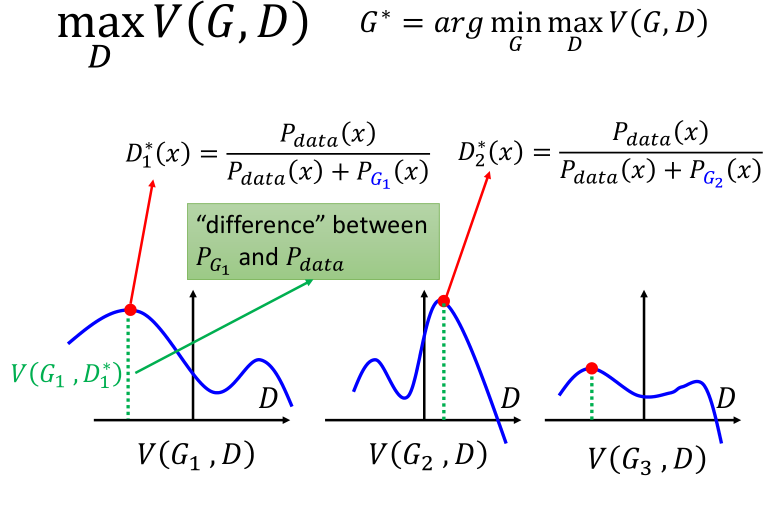#### 算法总结

1. 给定一个初始的 $G_0$ ；
2. 找到 $D_{0}^{* }$ ，最大化 $V(G_0,D)$ ;（这个最大化的过程其实就是最大化 $P_{data}(x)$ 和 $P_{G_0}(x)$ 的交叉熵的过程）
3. 使用梯度下降更新 $G$ 的参数 $θ_G := θ_G - \eta \frac{\partial\ \underset{D}{max}\ V(G,D_{0}^{* })}{\partial\ θ_G}$ ，得到 $G_1$；
4. 找到 $D_{1}^{* }$ ，最大化 $V(G_1,D)$ ;（这个最大化的过程其实就是最大化 $P_{data}(x)$ 和 $P_{G_1}(x)$ 的交叉熵的过程）
5. 使用梯度下降更新 $G$ 的参数 $θ_G := θ_G - \eta \frac{\partial\ \underset{D}{max}\ V(G,D_{1}^{* })}{\partial\ θ_G}$ ，得到 $G_2$；
6. 循环……

#### 实际情况下的算法总结

• 初始化一个 由 $θ_D$ 决定的 $D$ 和由 $θ_G$ 决定的 $G$；
• 循环迭代训练过程：
• 训练判别器（D）的过程，循环 $k$ 次：
• 从真实分布 $P_{data}(x)$ 中抽样 $m$个正例 $\lbrace x^{(1)},x^{(2)},x^{(3)},…,x^{(m)} \rbrace$
• 从先验分布 $P_{prior}(x)$ 中抽样 $m$个噪声向量 $\lbrace z^{(1)},z^{(2)},z^{(3)},…,z^{(m)} \rbrace$
• 利用生成器 $\tilde x^i = G(z^i)$ 输入噪声向量生成 $m$ 个反例 $\lbrace \tilde x^{(1)},\tilde x^{(2)},\tilde x^{(3)},…,\tilde x^{(m)} \rbrace$
• 最大化 $\tilde V$ 更新判别器参数 $θ_D$：
• $\tilde V = \frac{1}{m}\sum_{i=1}^{m} log D(x^i) + \frac{1}{m}\sum_{i=1}^{m} log (1-D(\tilde x^i))$
• $θ_D := θ_D - \eta \nabla \tilde V(θ_D)$
• 训练生成器（G）的过程，循环 $1$ 次：
• 从先验分布 $P_{prior}(x)$ 中抽样 $m$个噪声向量 $\lbrace z^{(1)},z^{(2)},z^{(3)},…,z^{(m)} \rbrace$
• 最小化 $\tilde V$ 更新生成器参数 $θ_G$：
• $\require{cancel}\tilde{V}=\cancel{\frac{1}{m}\sum_{i=1}^m\log D(x^i)}+\frac{1}{m}\sum_{i=1}^m\log(1-D(G(z^i)))$
• $θ_G := θ_G - \eta \nabla \tilde V(θ_G)$

## 关于最小化 V 以训练 G 的一点经验操作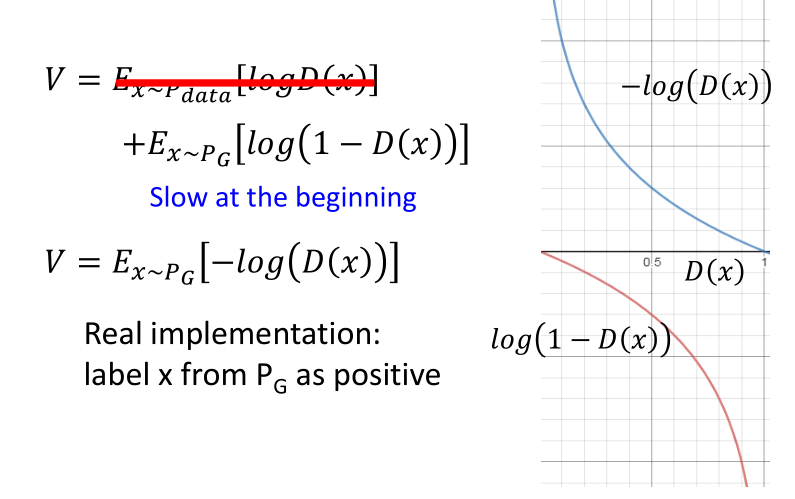## GAN训练的几个问题

#### 训练不稳定#### Mode Collapse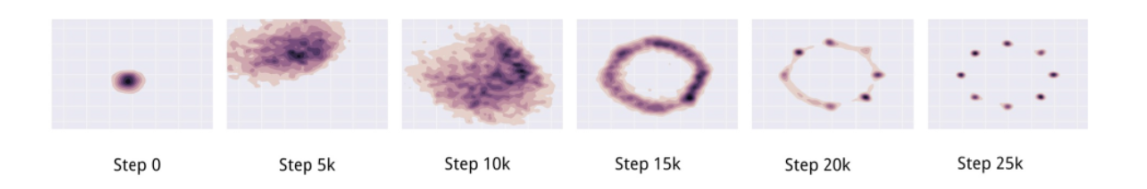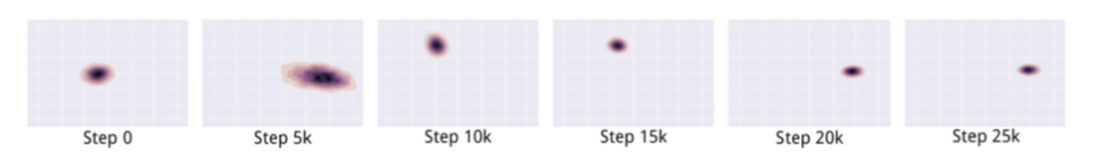本作品采用知识共享署名-相同方式共享 4.0 国际许可协议进行许可。欢迎转载，并请注明来自：黄钢的博客 ，同时保持文章内容的完整和以上声明信息！